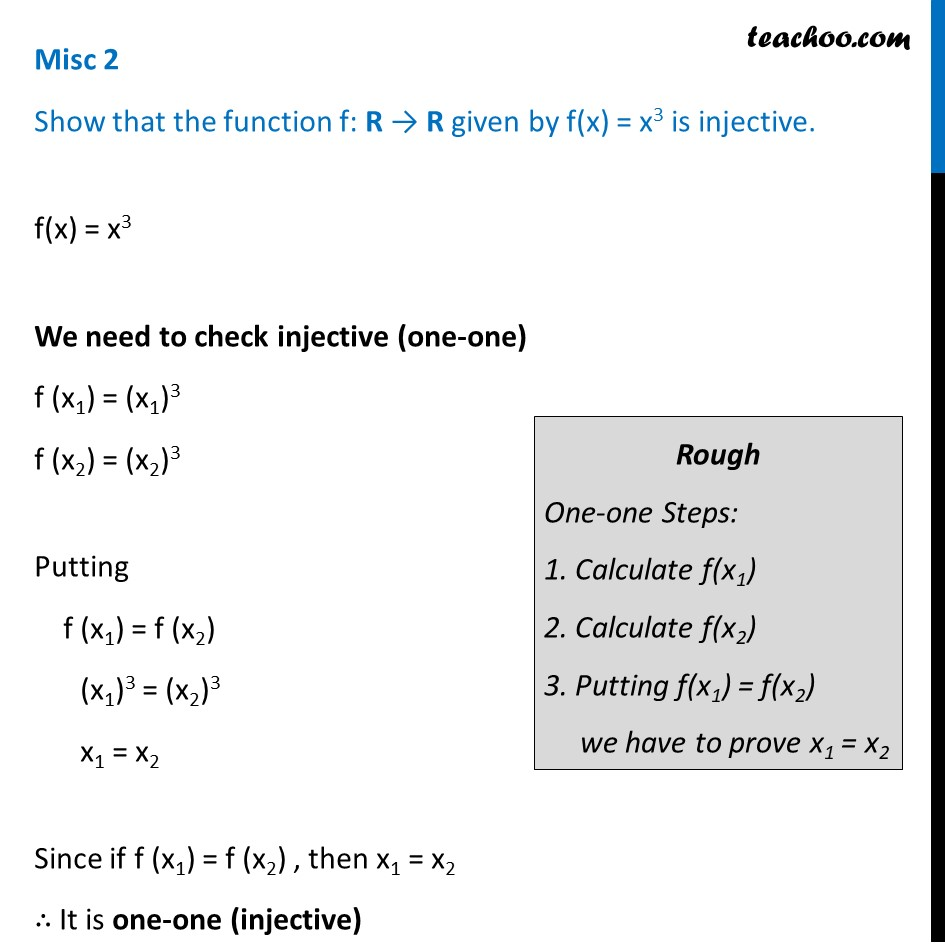To prove one-one & onto (injective, surjective, bijective)

Chapter 1 Class 12 Relation and Functions
Concept wiseLearn in your speed, with individual attention - Teachoo Maths 1-on-1 Class

### Transcript

Misc 2 Show that the function f: R → R given by f(x) = x3 is injective. f(x) = x3 We need to check injective (one-one) f (x1) = (x1)3 f (x2) = (x2)3 Putting f (x1) = f (x2) (x1)3 = (x2)3 x1 = x2 Since if f (x1) = f (x2) , then x1 = x2 ∴ It is one-one (injective) Rough One-one Steps: 1. Calculate f(x1) 2. Calculate f(x2) 3. Putting f(x1) = f(x2) we have to prove x1 = x2